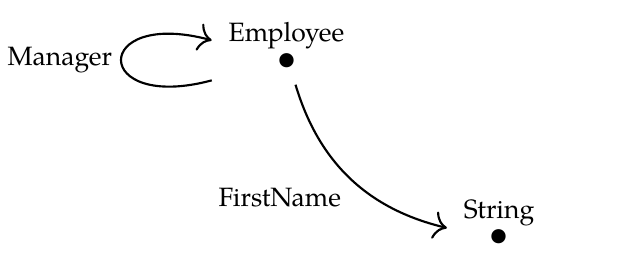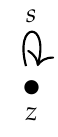We can do many things with databases using category theory. In fact David Spivak helps run a company called [Categorical Informatics](http://catinf.com/) that specializes in this! And his partner, [Ryan Wisnesky](https://forum.azimuthproject.org/discussion/2217/introduction-ryan-wisnesky/p1), would be happy to talk to people about it: click the link, he's attending this course. One important thing we can do is transform databases. Suppose we have a database schema \$$\mathcal{C}\$$. Remember, this is just a category. And suppose we have a database built using this schema: $F: \mathcal{C} \to \mathbf{Set} .$ Remember, this is just a functor. How can we we transform our database into a _different_ database built using a _different_ schema? There's an easy way and two harder, more interesting ways. Today I'll only talk about the easy way. Suppose we have another database schema \$$\mathcal{D}\$$: that is, another category. And suppose we have a functor $G : \mathcal{D} \to \mathcal{C} .$ Then we can turn our database $F: \mathcal{C} \to \mathbf{Set}$ into a new database by 'composing it' with \$$G\$$. The result is called $F \circ G : \mathcal{D} \to \mathbf{Set} .$ This is a database built using the schema \$$\mathcal{D}\$$. To understand this, you need to understand what it means to 'compose' functors. And, you need to think about some examples! Let's start with the first part. We will define composition of functors quite generally, not just for databases, because it's an incredibly important concept with tons of applications: **Definition.** Given functors \$$A: \mathcal{X} \to \mathcal{Y} \$$ and \$$B: \mathcal{Y} \to \mathcal{Z}\$$ there is a functor \$$B \circ A : \mathcal{X} \to \mathcal{Z}\$$, called the **composite** of \$$A\$$ and \$$B\$$, defined as follows: 1) For any object \$$x\$$ of \$$\mathcal{X}\$$ we have $(B \circ A)(x) = B(A(x)) .$ 2) For any morphism \$$f : x \to x'\$$ of \$$\mathcal{X}\$$ we have $(B \circ A)(f) = B(A(f)) .$ So, it's just what you'd guess! Of course you need to show that it's a functor. **Puzzle 119.** Show that if \$$A: \mathcal{X} \to \mathcal{Y} \$$ and \$$B: \mathcal{Y} \to \mathcal{Z}\$$ are functors, \$$B \circ A : \mathcal{X} \to \mathcal{Z}\$$ defined as above is really a functor. (Hint: see the definition of functor in [Lecture 38](https://forum.azimuthproject.org/discussion/2213/lecture-38-chapter-3-databases/p1) and check all the conditions.) Anything called 'composition' should be associative. Luckily, it's easy to check that composition of functors really is associative. It's so easy that I'll actually do it! Suppose we have three functors \$$A,B,C\$$ that can be composed. On objects we have $((C \circ B) \circ A)(x) = (C\circ B)(A(x)) = C(B(A(x)) = C((B\circ A)(x)) = (C \circ (B \circ A))(x) .$ We can do the same calculation for morphisms, too! A functor is determined by what it does to objects and morphisms. So, we have $(C \circ B) \circ A = C \circ (B \circ A) .$ Whenever you have something called 'composition', there should also be 'identities'. Indeed: **Definition.** For any category \$$\mathcal{X}\$$ there is an **identity functor** \$$1_\mathcal{X} : \mathcal{X} \to \mathcal{X}\$$, defined as follows: 1) For any object \$$x\$$ of \$$\mathcal{X}\$$ we have $1_{\mathcal{X}} (x) = x .$ 2) For any morphism \$$f : x \to x'\$$ of \$$\mathcal{X}\$$ we have $1_{\mathcal{X}} (f) = f .$ Again, it's just what you'd guess. By this point, you should be ready for this mind-blowing idea: **Definition.** There is a category \$$\mathbf{Cat}\$$ where: 1. the objects of \$$\mathbf{Cat}\$$ are categories, 2. if \$$\mathcal{X},\mathcal{X}'\$$ are sets, a morphism \$$F: \mathcal{X} \to \mathcal{X}'\$$ is a functor from \$$\mathcal{X}\$$ to \$$\mathcal{X}'\$$, and composition and identities are defined as above. The category of all categories!Logicians know this gives rise to paradoxes, just like [the set of all sets](https://en.wikipedia.org/wiki/Russell%27s_paradox). But it's easy to sidestep these, and it's not a big deal unless you're into this kind of thing, so I won't go into it now. I've spent years in the coal mines of mathematical logic, and it has its charm, but I'm trying to get to a point about databases here! _Namely_, we can take a database, which is just a functor: $F: \mathcal{C} \to \mathbf{Set}$ and take another functor: $G : \mathcal{D} \to \mathcal{C}$ and get a new database by composing them: $F \circ G : \mathcal{D} \to \mathbf{Set} .$ But _what does this actually do for us?_ Let's do an example! In [Lecture 36](https://forum.azimuthproject.org/discussion/2204/lecture-36-categories-from-graphs/p1) we saw how to turn any graph into a category, the 'free category' on that graph. So let's create a category \$$\mathcal{C}\$$ from this graph:and imagine we have a database \$$F: \mathcal{C} \to \mathbf{Set} \$$, which tells us an actual set of employees, an actual set of departments, an actual function mapping each employee to their department, etc. Then, let's create a new category \$$\mathcal{D}\$$ from a smaller graph that forgets everything about the departments:This is a 'subgraph' of our earlier graph: simply put, it sits inside that bigger graph! So, we get a functor $G : \mathcal{D} \to \mathcal{C}$ which does this on objects: $G(\textrm{Employee}) = \textrm{Employee}$ $G(\textrm{String}) = \textrm{String}$ and this on morphisms: $G(\textrm{Manager}) = \textrm{Manager}$ $G(\textrm{FirstName}) = \textrm{FirstName} .$ Boring, huh? It looks almost like an identity functor, but it's not, because \$$\mathcal{D}\$$ has more objects and morphisms. We say that \$$\mathcal{C}\$$ is a [subcategory](https://en.wikipedia.org/wiki/Subcategory) of \$$\mathcal{D}\$$. Now, we can compose \$$F\$$ and \$$G\$$ and get a new database: $F \circ G : \mathcal{D} \to \mathbf{Set} .$ What is this like? Simple! It's just like our original database $F : \mathcal{C} \to \mathbf{Set}$ except it forgets all the data involving departments! If you don't see this, please draw an example. In short, we've taken a database and 'trimmed it down' to a smaller database by leaving out some objects and morphisms in our original database schema. We can also do other things using composition: **Puzzle 120.** Take a database \$$F: \mathcal{C} \to \mathbf{Set}\$$ and some functor \$$H : \mathcal{E} \to \mathcal{C} \$$ that is not one-to-one on objects and morphisms, and see what the resulting database \$$F \circ H : \mathcal{E} \to \mathbf{Set} \$$ looks like. There are also millions of other things to say about functors. Just to give you a little practice: **Puzzle 121.** Let \$$\mathbf{N}\$$ be the free category on this graph:It has one morphism for each natural number. What are all the functors \$$F: \mathbf{N} \to \mathbf{N}\$$? What happens when we compose them? **[To read other lectures go here.](http://www.azimuthproject.org/azimuth/show/Applied+Category+Theory#Chapter_3)**